# KMP算法的实现及其优化

## April 8, 2020 • 算法

kmp算法的实现有多种方式，我这里讲两种：

## kmp算法简介

KMP 算法是一种改进的字符串匹配算法，由 D.E.Knuth，J.H.Morris 和 V.R.Pratt 提出的，因此人们称它为克努特莫里斯普拉特操作（简称 KMP 算法）。KMP 算法的核心是利用匹配失败后的信息，尽量减少模式串与主串的匹配次数以达到快速匹配的目的。具体实现就是通过一个 next () 函数实现，函数本身包含了模式串的局部匹配信息。KMP 算法的时间复杂度 O(m+n)。 ——摘自百度百科

``````/*
* @Author: Mr.Sen
* @LastEditTime: 2020-04-08 13:43:16
* @Website1: 449293786.site
*/
#include<bits/stdc++.h>
using namespace std;
int search(string cmp,string pattern)
{
int i=0,j=0;
while (i<cmp.length()&&j<pattern.length())
{
if (cmp[i]==pattern[j])
{
i++,j++;
}
else
{
i=i-j+1;
j=0;
}
}
if (j=pattern.length())
{
return i-j+1;
}
return -1;
}
int main()
{
string cmp,pattern;
cin>>cmp>>pattern;
cout<<search(cmp,pattern)<<endl;
return 0;
}``````

## 一、传统写法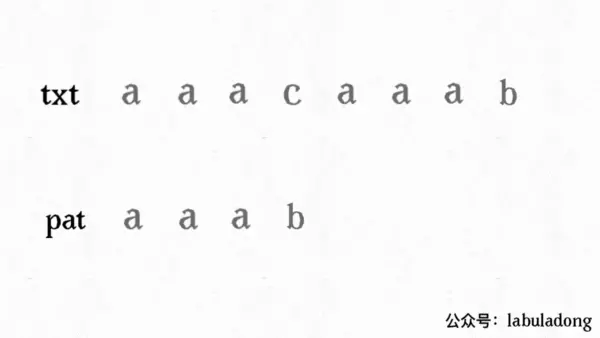### search()函数

search函数和暴力写法比较类似

``````/*
* @Author: Mr.Sen
* @LastEditTime: 2020-04-08 13:10:56
* @Website1: 449293786.site
*/
int search(string cmp, string pattern)
{
if(pattern == "")
return 0;
int* next = get_next(pattern);
int i=0;//待匹配字符串指针
int j=0;//模式字符串指针
while(i <cmp.length() && j <pattern.length())
{
if(j == -1 || cmp[i] == pattern[j])
{
i++;j++;
//匹配成功或者模式指针被回退到了-1
}
else
{
j = next[j];
//匹配失败，模式指针回退到next[j]
}
}
if(j == pattern.length())
{
//模式指针走完全程，匹配成功
return i-j;
}
else
{
//匹配失败，返回-1
return -1;
}
}``````

### next数组

i为待匹配字符串指针，j为模式字符串指针

#### next数组怎么计算？

##### 情形1：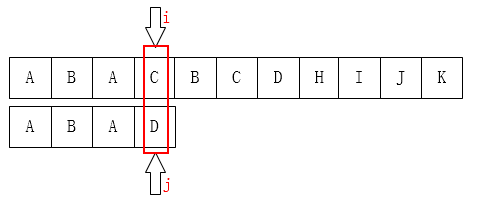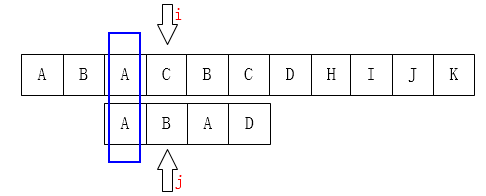##### 情形2：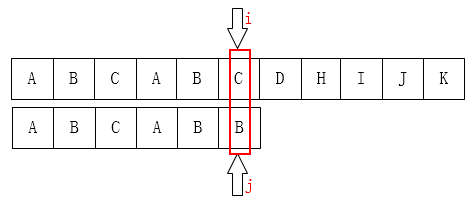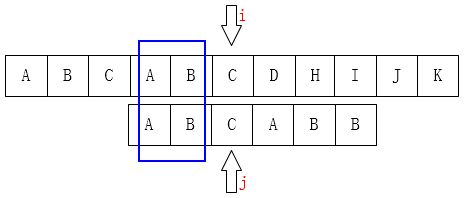ABCABB，其中发生失配的B前面，有ABCAB，其中AB和AB是一样的，长度为2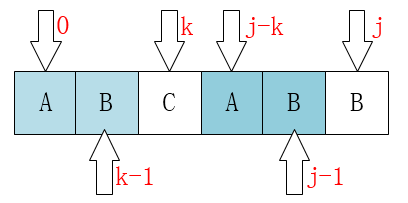ABABABB -> -1 0 0 1 2 3 4
ABAB -> -1 0 0 1
AAAB -> -1 0 1 2

#### 接下来的问题就是如何利用代码计算next数组了

``````/*
* @Author: Mr.Sen
* @LastEditTime: 2020-04-08 14:52:57
* @Website1: 449293786.site
*/
#include <bits/stdc++.h>
using namespace std;
int* get_next(string pattern)
{
int* next = new int[pattern.length()];
//动态申请一段空间
next = -1;
//将next数组初始化
int i = 0;//模式字符串指针
int k = -1;//记录最大公共前缀长度
int size = pattern.length();
while(i<size - 1)
{
if(k == -1 || pattern[k] == pattern[i])
{
//如果匹配上了，公共前缀长度增加
i++;k++; next[i] = k;
}
else
{
//如果失配了，改变公共前缀的长度
k = next[k];
}
}
return next;
}

int main()
{
string pattern;
cin>>pattern;
int *next=get_next(pattern);
for (int i=0;i<pattern.length();i++)
{
cout<<next[i]<<" ";
}
return 0;
}``````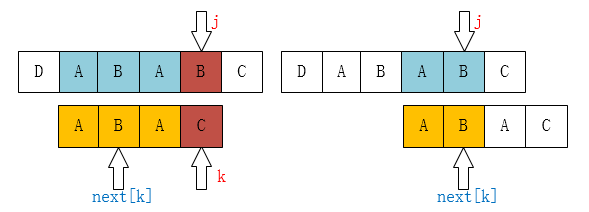#### 传统版本代码：

``````/*
* @Author: Mr.Sen
* @LastEditTime: 2020-04-09 09:39:32
* @Website1: 449293786.site
*/
#include <bits/stdc++.h>
using namespace std;
int* get_next(string pattern)
{
int* next = new int[pattern.length()];
next = -1;
int j = 0;
int k = -1;
int size = pattern.length();
while(j<size - 1)
{
if(k == -1 || pattern[k] == pattern[j])
{
j++;k++; next[j] = k;
}
else
{
k = next[k];
}
}
return next;
}
int KMP(string cmp, string pattern)
{
if(pattern == "") return 0;
int* next = get_next(pattern);
int i=0;
int j=0;
while(i < int(cmp.length()) && (j < int(pattern.length())))
{
if(j == -1 || cmp[i] == pattern[j])
{
i++;j++;
}
else
{
j = next[j];
}
}
if(j == pattern.length())
{
return i-j;
}
else
{
return -1;
}
}
int main()
{
string cmp,pattern;
cin>>cmp>>pattern;
int location=KMP(cmp,pattern);
cout<<location<<endl;
return 0;
}``````
##### 传统代码分析：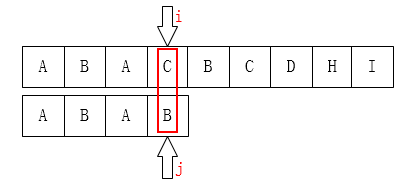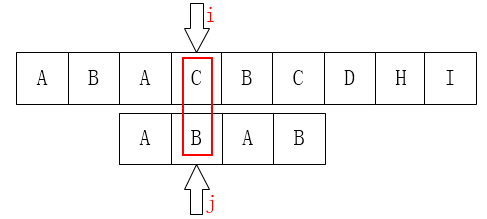#### 传统代码升级版

``````/*
* @Author: Mr.Sen
* @LastEditTime: 2020-04-08 12:31:30
* @Website1: 449293786.site
*/
#include<bits/stdc++.h>
using namespace std;
int* get_next(string pattern)
{
//get next array and return a point
int size=pattern.length();
int* next=new int[size];
//动态申请一块空间
next=-1;
//给零号位初始化为-1，当零号位不能匹配时整体后移一位
int j=0,k=-1;
while (j<pattern.length()-1)
{
if (k==-1||pattern[j]==pattern[k])
{
if (pattern[++j]==pattern[++k])
{
next[j]=next[k];
}
else next[j]=k;
}
else k=next[k];
}
return next;
}

int KMP(string cmp, string pattern)
{
if(pattern == "")
return 0;
int* next = get_next(pattern);
int i=0;
int j=0;
int cnt=0;
while(i < int(cmp.length()) && (j < int(pattern.length())))
{
if(j == -1 || cmp[i] == pattern[j])
{
i++;j++;
}
else
{
j = next[j];
}
cnt++;
}
cout<<"cnt="<<cnt<<endl;
if(j == pattern.length())
{
return i-j;
}
else
{
return -1;
}
}

int main()
{
string cmp,pattern;
int number1,number2;
cin>>cmp>>pattern;
cout<<KMP(cmp,pattern);
return 0;
}``````

## 二、确定有限状态机

``````void get_next(string pattern)
{
//通过pattern构建next数组
}
void kmp(string cmp)
{
//借助next数组来匹配cmp
}``````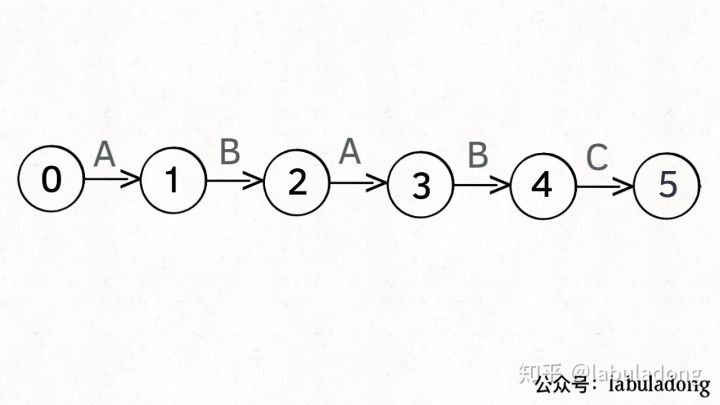#### 接下来我们看几个例子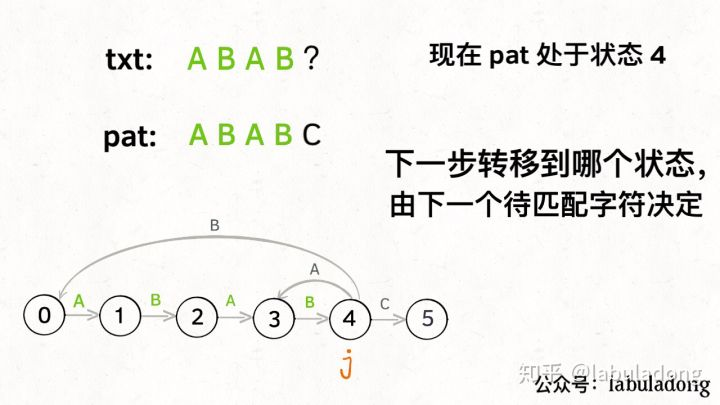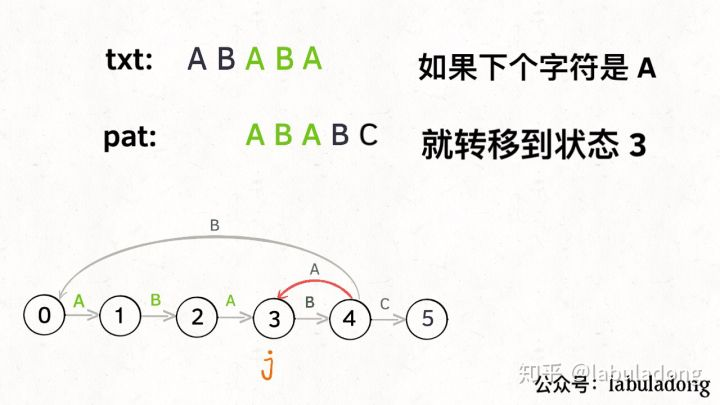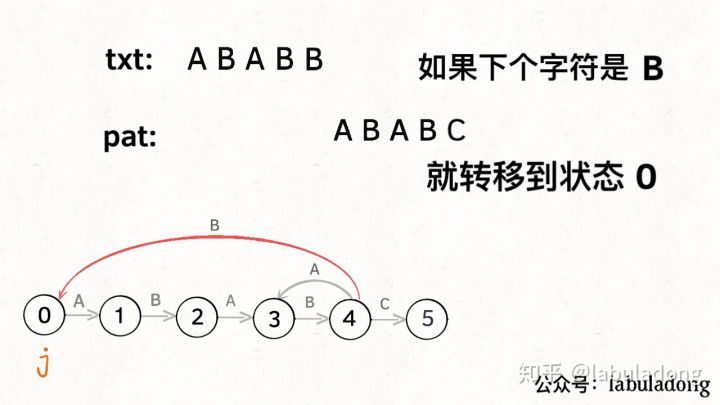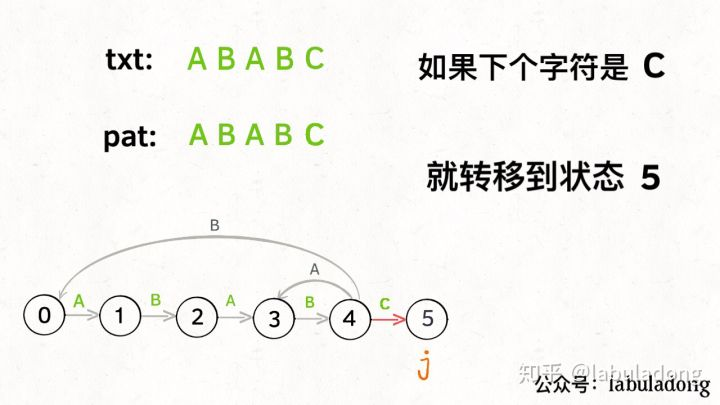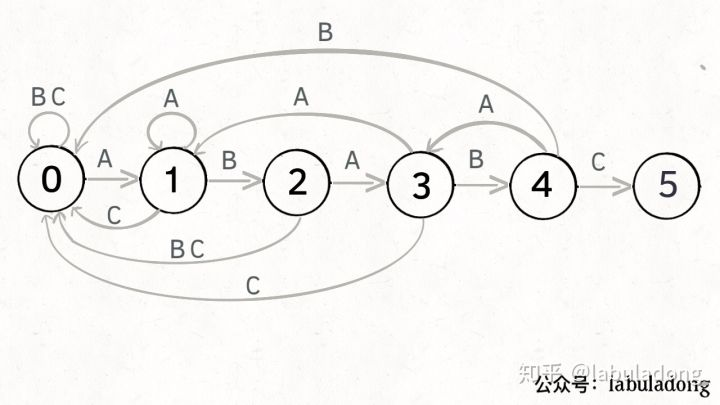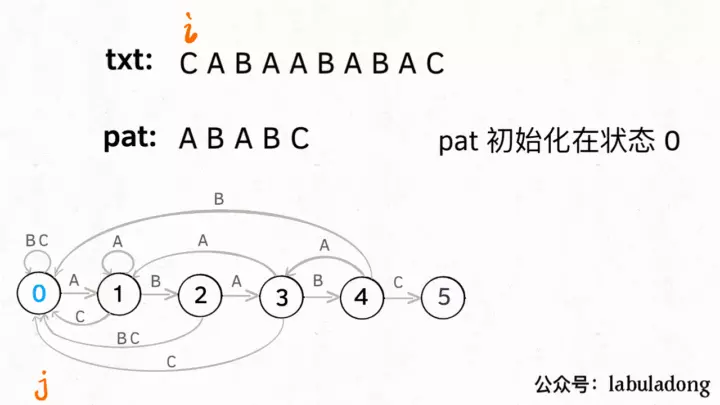#### next数组怎么计算？

``````next[j][c]=next_status
0 <= j < M，代表当前的状态
0 <= c < 256，代表遇到的字符（ASCII 码）
0 <= next_status <= M，代表下一个状态

next['A'] = 3 表示：

patttern 应该转移到状态 3

next['B'] = 2 表示：

patttern 应该转移到状态 2``````

``````for 0 <= j < M: # 状态
for 0 <= c < 256: # 字符
dp[j][c] = next``````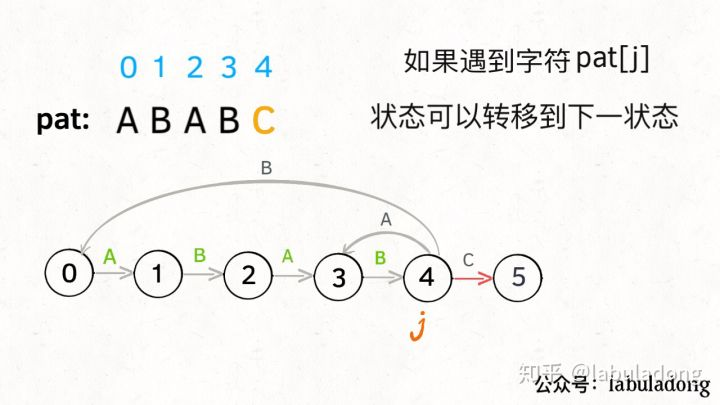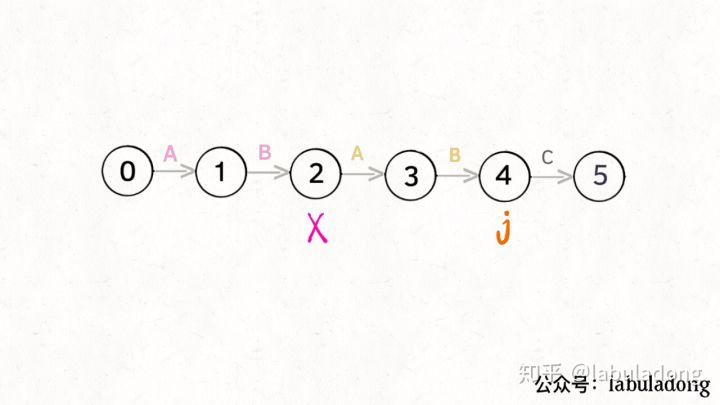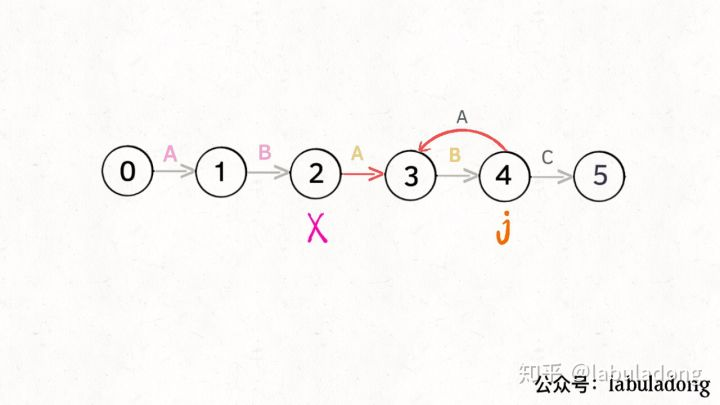kmp中，为了减少不必要的计算，j就应该去找和自己有相同前缀的x：如果x遇见“A”，该回退到哪个位置。这样转移过去，才能使回退最少，j只需要跟着x走就行了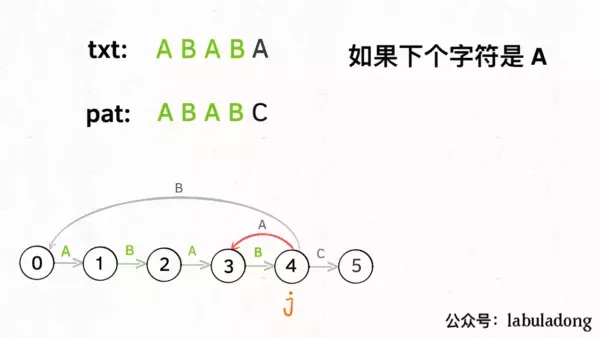``````int X # 影子状态
for 0 <= j < M:
for 0 <= c < 256:
if c == pat[j]:
# 状态推进
dp[j][c] = j + 1
else:
# 状态重启
# 委托 X 计算重启位置
dp[j][c] = dp[X][c]``````

#### 最后，给出确定有限状态机版本的方程：

``````/*
* @Author: Mr.Sen
* @LastEditTime: 2020-04-08 13:08:01
* @Website1: 449293786.site
*/
#include<bits/stdc++.h>
using namespace std;
int next1;
void get_next(string pattern)
{
int size=pattern.length();
next1[pattern]=1;
for (int i=1;i<size;i++)
{
for (int j=0;j<256;j++)
{
}
next1[i][pattern[i]]=i+1;
}
}
int search(string cmp,string pattern)
{
int size_pattern=pattern.length();
int size_cmp=cmp.length();
int j=0;
for (int i=0;i<cmp.length();i++)
{
j=next1[j][cmp[i]];
if (j==size_pattern)
return i-size_pattern+1;
}
return -1;
}
int main()
{
get_next("sip");
cout<<search("missisasipi","sip");
return 0;
}``````

https://zhuanlan.zhihu.com/p/83334559

https://www.cnblogs.com/yjiyjige/p/3263858.html